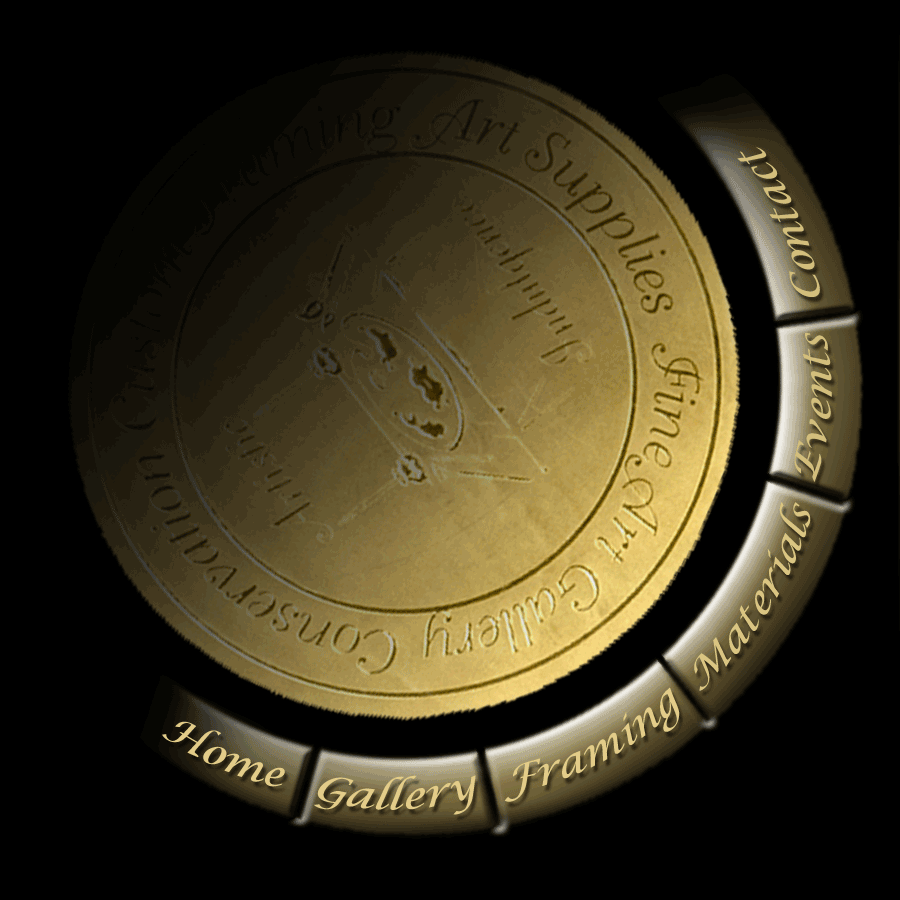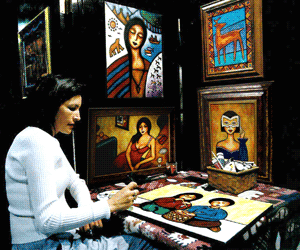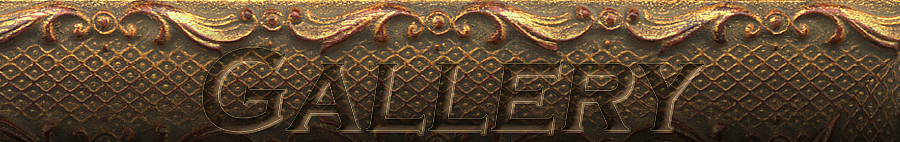" Where Your Art Matters "

Artist Owned and Operated since 1996# Mary Howard Figurative Artist of Feminine Sensuality

Back to Mary's Gallery

Available Pre - Painted Originals
Commissioned Originals

5 X 7 = \$150

8 X 10 = \$300

9 X 12 = \$400

11 X 14 = \$475

12 X 16 = \$550

14 X 17 = \$625

16 X 20 = \$750

18 X 24 = \$850

20 X 24 = \$950

22 X 28 = \$1400

24 X 36 = \$2000

24 X 48 = \$3000

5 X 7 = \$225

8 X 10 = \$400

9 X 12 = \$500

11 X 14 = \$600

12 X 16 = \$700

14 X 17 = \$800

16 X 20 = \$950

18 X 24 = \$1050

20 X 24 = \$1400

22 X 28 = \$2000

24 X 36 = \$3000

24 X 48 = \$4000

Prices are subject to change, and prices in the pre-painted category may differ depending on possible existing frame, additional work done to painting or odd size.

Back to Mary's Gallery